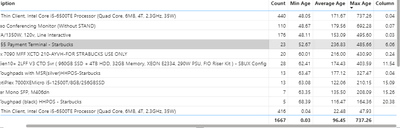cancel
Showing results for
Did you mean:

Fabric is Generally Available. Browse Fabric Presentations. Work towards your Fabric certification with the Cloud Skills Challenge.Frequent Visitor

## Creating columns for count, min, avg, and max calculations based on parameter from another column

I have a table with count, min, avg, and max for each item, however I also want to show count, min, avg, and max for items with age >=48 hours in the same table. For reference Age column has values:Power BI does not allow me to set a filter on a specific column (applies to entire visual) so it affects the previous count, min, avg, and max calculations that I want to keep separate. I tried creating a calculated column for the minimum when age >=48 hours:

Column = IF('NCR Open Calls'[Age Sort]="less than 48",BLANK(),CALCULATE(MIN('NCR Open Calls'[AGE]),FILTER('NCR Open Calls','NCR Open Calls'[ITEM DESCRIPTION]=EARLIER('NCR Open Calls'[ITEM DESCRIPTION]))))

However, I am not getting data I would expect.Any improvements for the logic or formula would be appreciated!

1 ACCEPTED SOLUTIONSolution Sage

You can start creating measures for the new count, min, avg, and max calculations that consider only items with age >= 48 hours:

``````CountAgeGreater48 =
CALCULATE(
COUNT('NCR Open Calls'[AGE]),
FILTER(
'NCR Open Calls',
'NCR Open Calls'[Age Sort] <> "less than 48"
)
)``````

Then Min for Age >= 48 hours:

``````MinAgeGreater48 =
CALCULATE(
MIN('NCR Open Calls'[AGE]),
FILTER(
'NCR Open Calls',
'NCR Open Calls'[Age Sort] <> "less than 48"
)
)``````

Then Average for Age >= 48 hours:

``````AvgAgeGreater48 =
CALCULATE(
AVERAGE('NCR Open Calls'[AGE]),
FILTER(
'NCR Open Calls',
'NCR Open Calls'[Age Sort] <> "less than 48"
)
)``````

And finally Max for Age >= 48 hours:

``````MaxAgeGreater48 =
CALCULATE(
MAX('NCR Open Calls'[AGE]),
FILTER(
'NCR Open Calls',
'NCR Open Calls'[Age Sort] <> "less than 48"
)
)``````

Now, when you use these measures in a table visual, they will automatically take the context of the visual into account. This means that, for each item description, Power BI will only consider rows with age >= 48 hours when computing the values of these measures.

This approach ensures that other calculations (your original count, min, avg, max) remain unaffected by the >= 48 Note that if `'NCR Open Calls'[Age Sort]` is a derived column from `'NCR Open Calls'[AGE]`, you can also directly filter based on the AGE column value itself. For instance, if AGE is in hours, you can replace `'NCR Open Calls'[Age Sort] <> "less than 48"` with `'NCR Open Calls'[AGE] >= 48` in the measures above.

Proud to be a Power BI Super User !

Microsoft Community : https://docs.microsoft.com/en-us/users/AmiraBedhiafi
StackOverflow : https://stackoverflow.com/users/9517769/amira-bedhiafi
C-Sharp Corner : https://www.c-sharpcorner.com/members/amira-bedhiafi
Power BI Community :https://community.powerbi.com/t5/user/viewprofilepage/user-id/332696Solution Sage

You can start creating measures for the new count, min, avg, and max calculations that consider only items with age >= 48 hours:

``````CountAgeGreater48 =
CALCULATE(
COUNT('NCR Open Calls'[AGE]),
FILTER(
'NCR Open Calls',
'NCR Open Calls'[Age Sort] <> "less than 48"
)
)``````

Then Min for Age >= 48 hours:

``````MinAgeGreater48 =
CALCULATE(
MIN('NCR Open Calls'[AGE]),
FILTER(
'NCR Open Calls',
'NCR Open Calls'[Age Sort] <> "less than 48"
)
)``````

Then Average for Age >= 48 hours:

``````AvgAgeGreater48 =
CALCULATE(
AVERAGE('NCR Open Calls'[AGE]),
FILTER(
'NCR Open Calls',
'NCR Open Calls'[Age Sort] <> "less than 48"
)
)``````

And finally Max for Age >= 48 hours:

``````MaxAgeGreater48 =
CALCULATE(
MAX('NCR Open Calls'[AGE]),
FILTER(
'NCR Open Calls',
'NCR Open Calls'[Age Sort] <> "less than 48"
)
)``````

Now, when you use these measures in a table visual, they will automatically take the context of the visual into account. This means that, for each item description, Power BI will only consider rows with age >= 48 hours when computing the values of these measures.

This approach ensures that other calculations (your original count, min, avg, max) remain unaffected by the >= 48 Note that if `'NCR Open Calls'[Age Sort]` is a derived column from `'NCR Open Calls'[AGE]`, you can also directly filter based on the AGE column value itself. For instance, if AGE is in hours, you can replace `'NCR Open Calls'[Age Sort] <> "less than 48"` with `'NCR Open Calls'[AGE] >= 48` in the measures above.

Proud to be a Power BI Super User !

Microsoft Community : https://docs.microsoft.com/en-us/users/AmiraBedhiafi
StackOverflow : https://stackoverflow.com/users/9517769/amira-bedhiafi
C-Sharp Corner : https://www.c-sharpcorner.com/members/amira-bedhiafi
Power BI Community :https://community.powerbi.com/t5/user/viewprofilepage/user-id/332696Announcements#### Power BI Monthly Update - November 2023

Check out the November 2023 Power BI update to learn about new features.#### Fabric Community News unified experience

Read the latest Fabric Community announcements, including updates on Power BI, Synapse, Data Factory and Data Activator.#### The largest Power BI and Fabric virtual conference

130+ sessions, 130+ speakers, Product managers, MVPs, and experts. All about Power BI and Fabric. Attend online or watch the recordings.Top Solution Authors
Top Kudoed Authors
Users online (2,950)